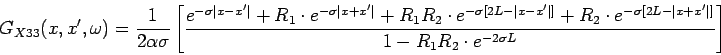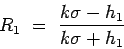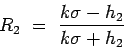X11 Plate, with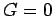(Dirichlet) at x=0 and at x=L.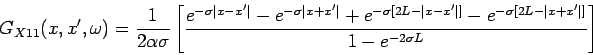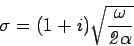X12 Plate, with(Dirichlet) at x=0 and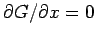(Neumann) at x=L.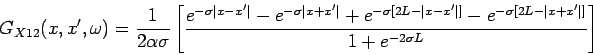X13 Plate, with(Dirichlet) at x=0 and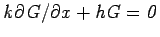(Convection) at x=L.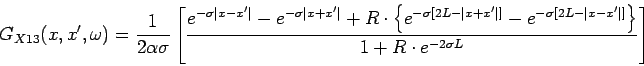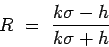X21 Plate, with(Neumann) at x=0 and(Dirichlet) at x=L.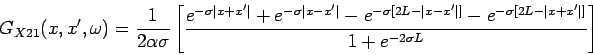X22 Plate, with(Neumann) at x=0 and at x=L.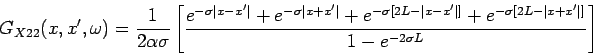X23 Plate, with(Neumann) at x=0 and(Convection) at x=L.X31 Plate, with(Convection) at x=0 and(Dirichlet) at x=L.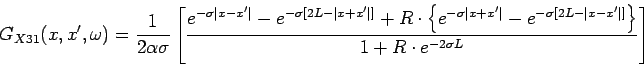X32 Plate, with(Convection) at x=0 and(Neumann) at x=L.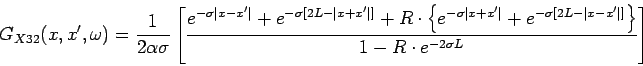X33 Plate, with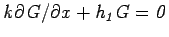(Convection) at x=0 and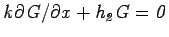(Convection) at x=L.